This topic describes why the instances of an auto triggered node are not run. This topic corresponds to the fourth question in the Node instance status topic.

## Instance status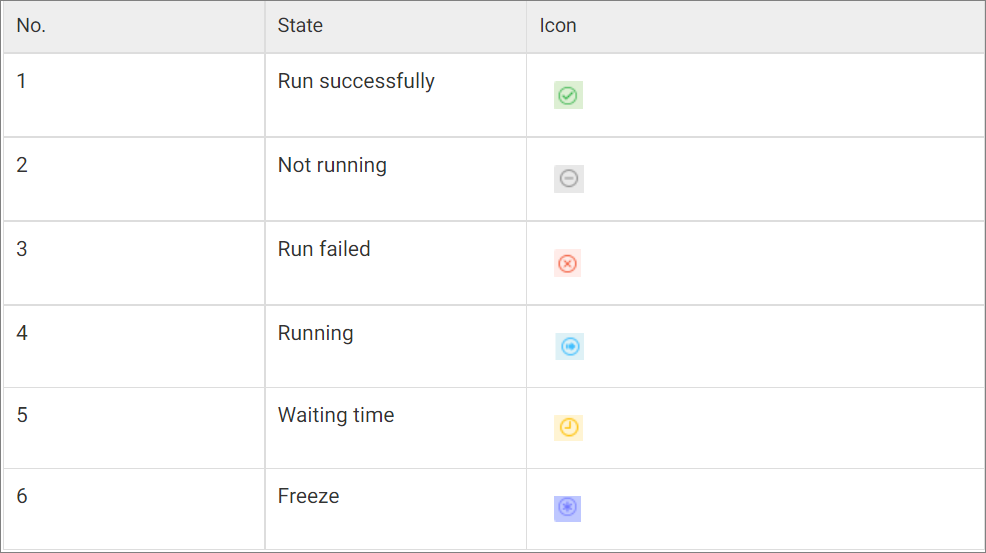## Why is the auto triggered node not run when the scheduled time arrives?

In some cases, the time at which an auto triggered instance is scheduled to run may not be the same as the time at which the instance is actually run.

In this case, we recommend that you use the ancestor node analysis and intelligent diagnosis features to identify and fix issues at the earliest opportunity.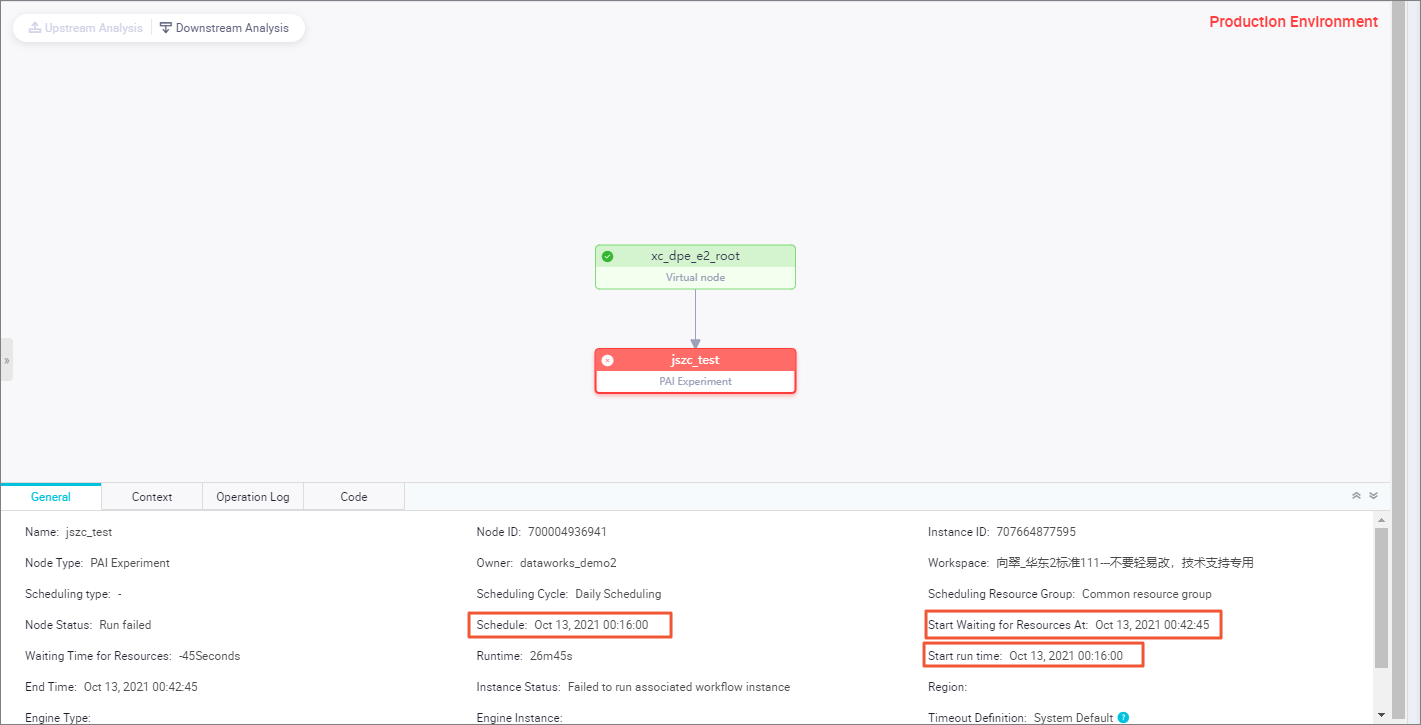The following conditions must be met if you want to run a node in DataWorks:
Notice The following figure shows a three-level node dependency. In actual scenarios, you may need to configure more than three levels of nodes in a dependency. However, the logic of the dependency is the same. You can right-click a dimmed node and select Show Ancestor Nodes to view the status of each ancestor node of the node.
1. All the ancestor nodes of the current auto triggered node have finished running. A node that is successfully run is marked in green. You can view the status of each ancestor node of the auto triggered node in a directed acyclic graph (DAG) in Operation Center.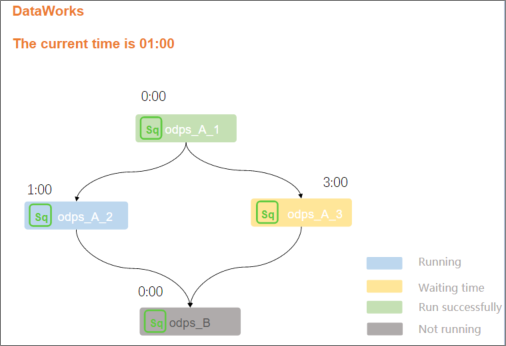Example: The current time is 01:00. Node A2 is scheduled to be run at 01:00, Node A3 is scheduled to be run at 03:00, and Node B is scheduled to be run at 00:00. Node B depends on Nodes A2 and A3.

Analysis: The time at which Node B is scheduled to be run has arrived. However, the time at which the ancestor nodes of Node B are scheduled to be run has not arrived. In this case, Node B cannot be run until the scheduled time of the ancestor nodes A2 and A3 arrives and the ancestor nodes A2 and A3 are successfully run. If Node A2 or Node A3 fails to be run as scheduled, Node B cannot be run as scheduled.

2. The scheduled time of the node has arrived. A node that is waiting for its scheduled time to arrive is marked in yellow.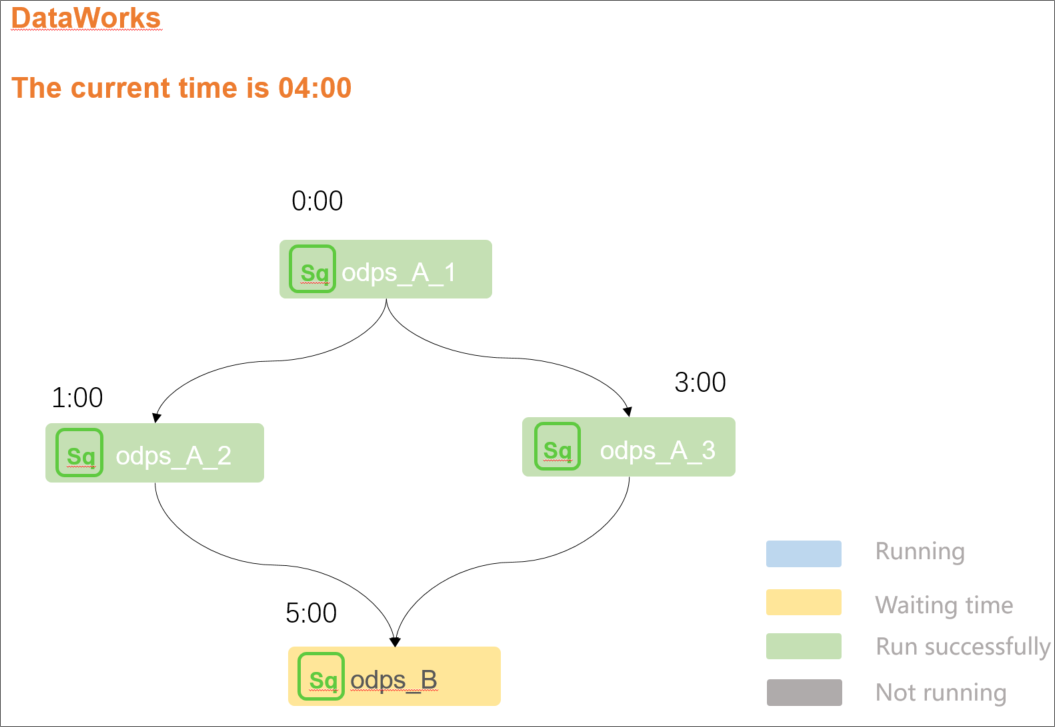Example: The current time is 04:00. Node A2 is scheduled to run at 01:00, Node A3 is scheduled to run at 03:00, and Node B is scheduled to run at 05:00. Node B depends on Nodes A2 and A3.

Analysis: Node B is scheduled to be run at 05:00 and Nodes A2 and A3 are successfully run. However, the time at which Node B is scheduled to be run has not arrived and therefore the instance status of Node B is waiting for its scheduled time to arrive.

3. The scheduling resources in a workspace are sufficient to run nodes. An auto triggered node that is waiting for scheduling resources is marked in yellow. This is because the number of auto triggered nodes that are run by using the scheduling resources in a workspace has reached the upper limit and the current node is waiting for gateway resources.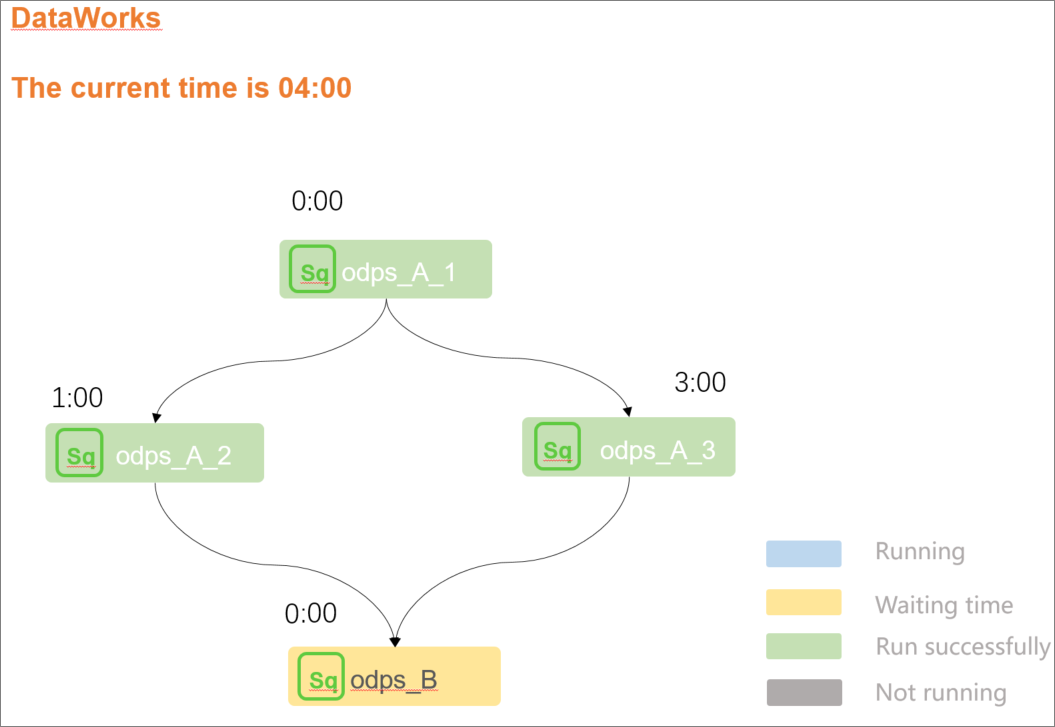Example: The current time is 04:00. Node A2 is scheduled to run at 01:00, Node A3 is scheduled to run at 03:00, and Node B is scheduled to run at 00:00. Node B depends on Nodes A2 and A3.

Analysis: Node B is scheduled to be run at 00:00 and Nodes A2 and A3 are successfully run. However, the scheduling resources that are used to run Node B are insufficient. As a result, the instance status of Node B is waiting for scheduling resources.

If Node B is run by using an exclusive resource group for scheduling and you want to view the resource usage of this group, you can use one of the following methods: 1. Log on to the DataWorks console. On the Resource Groups page, view the nodes that run on the exclusive resource group for scheduling and the usage of this resource group. For more information, see View the resource usage of an exclusive resource group. 2. Right-click an instance and select Instance Diagnose to check the nodes that are occupying resources in the exclusive resource group for scheduling.

4. The node is normally run as scheduled and is not frozen. A node that is frozen is marked in purple.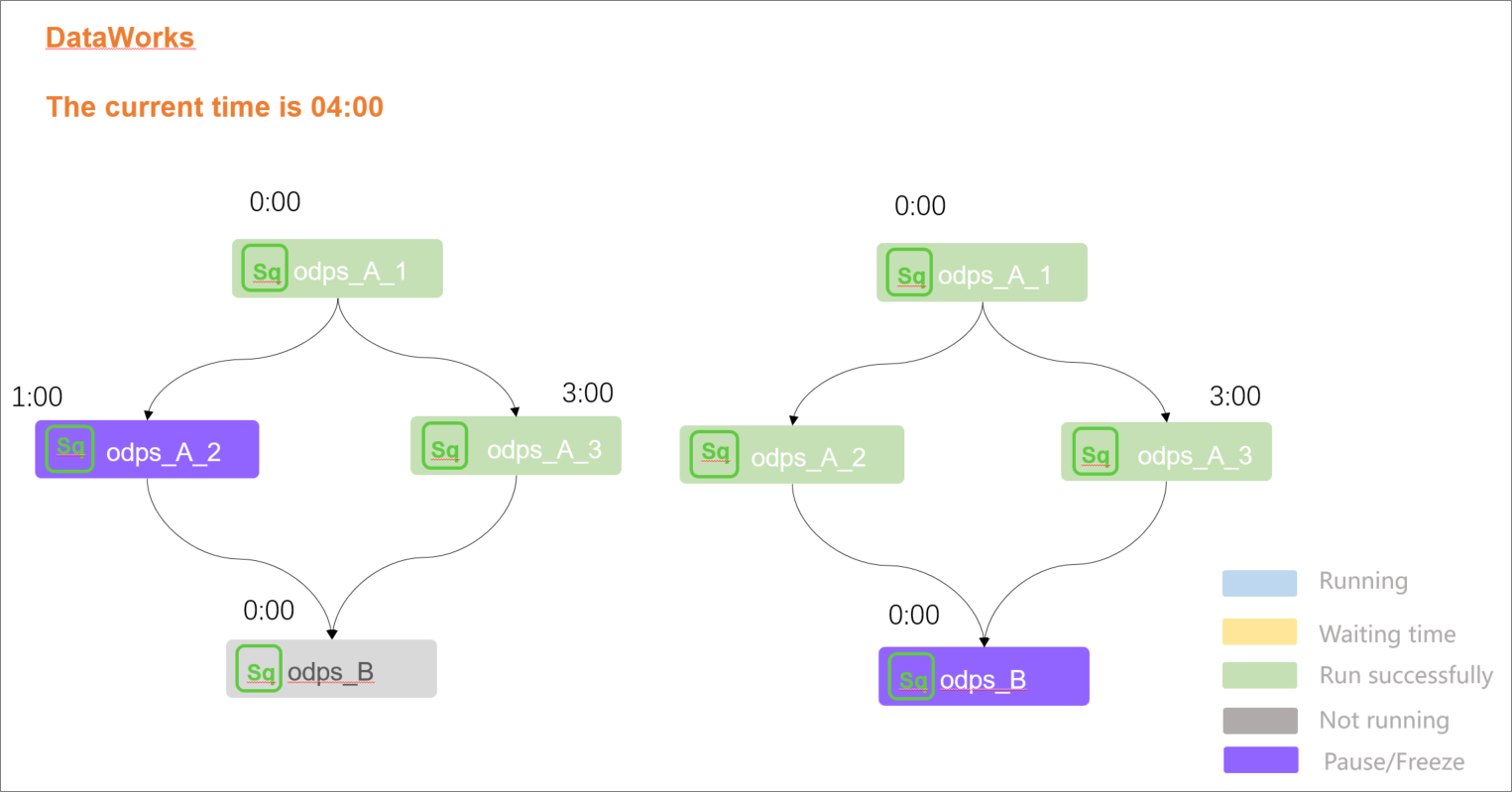Example: The current time is 04:00. Node A2 is scheduled to run at 01:00 and its status is scheduling suspended, Node A3 is scheduled to run at 03:00, and Node B is scheduled to run at 00:00. Node B depends on Nodes A2 and A3.

Analysis: The preceding information indicates that a node can be run as scheduled only after all of its ancestor nodes are successfully run. The preceding figure on the left shows that Node A2 is frozen. As a result, Node B cannot be run. The preceding figure on the right shows that all the ancestor nodes of Node B are successfully run but Node B cannot be run as scheduled. This is because Node B is frozen.